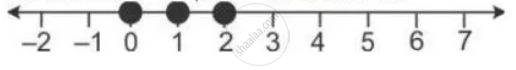Share

Books Shortlist

# Solve the Inequation -2 1/2 + 2x <= (4x)/5 <= 4/3 + 2x , X ∈ W. Graph the Solution Set on the Number Line. - ICSE Class 10 - Mathematics

ConceptRepresentation of Solution on the Number Line

#### Question

Solve the inequation

-2 1/2 + 2x <= (4x)/5 <= 4/3 + 2x , x ∈ W.

Graph the solution set on the number line.

#### Solution

-2 1/2 + 2x  <=  (4x)/5 <= 4/3 + 2x

-2 1/2 <=  (4x)/5  -  2x  <=   4/3

-5/2 <= - (6x)/5 <= 4/3

25/12 >= x  >= - 10/9

2.083 >= x >= -1.111

Since x ∈ W

∴ Solution set = {0, 1, 2}

The solution set can be represented on number line as:Is there an error in this question or solution?

#### APPEARS IN

Solution Solve the Inequation -2 1/2 + 2x <= (4x)/5 <= 4/3 + 2x , X ∈ W. Graph the Solution Set on the Number Line. Concept: Representation of Solution on the Number Line.
S